In this tutorial we’ll use the ANES data we used in Tutorial 2. Read in the file and clean it like we did in the Preliminaries section of that tutorial.

You should then have:

dim(anes)
##  49760    12

Also make sure you load the following packages:

libs <- c("plyr", "dplyr", "ggplot2", "arm")
sapply(libs, require, character.only = TRUE)
##    plyr   dplyr ggplot2     arm
##    TRUE    TRUE    TRUE    TRUE

The only package we haven’t used before is arm. It allows us to display OLS output more cleanly and to extract standard errors.

1 Interpreting and Graphing OLS results

Make note of two graphing approaches of OLS results below:

1. Graphing the coefficient estimates themselves with confidence intervals
2. Graphing predicted/fitted values with confidence intervals

1.1 Bivariate Model

Let’s start by a simple model that predicts democratic feeling ratings given the respondent’s gender.

levels(anes\$gender) <- c(NA, "Male", "Female")

lm1 <- lm(demtherm ~ gender, data = anes)

require(arm)    #for display function
display(lm1)
## lm(formula = demtherm ~ gender, data = anes)
##              coef.est coef.se
## (Intercept)  58.99     0.22
## genderFemale  5.65     0.29
## ---
## n = 26405, k = 2
## residual sd = 23.74, R-Squared = 0.01

How do we interpret the output? Compare the output with:

ddply(anes, .(gender), summarise, mean(demtherm, na.rm = T))
##   gender mean(demtherm, na.rm = T)
## 1   Male                     58.99
## 2 Female                     64.64
## 3   <NA>                       NaN

Does this make sense?

Graphing can aid in the interpretation of OLS estimates. The benefits to graphing aren’t obvious in this simple model, but let’s use it for illustrative purposes. We’ll use two approaches.

1.1.1 Graphing Approach 1

We can extract the coefficients from the model using coef() and the standard errors using se.coef(). Using these we can calculate confidence intervals and graph the results. We can also use confint() to find the confidence intervals directly.

ci1 <- coef(lm1) + c(-1, 1) * se.coef(lm1) * 1.96   #or equivalently:
ci1 <- confint(lm1, level = 0.95)[2, ]

est1 <- data.frame(est = coef(lm1),
lb = ci1,
ub = ci1,
model = "Model 1")

ggplot(est1, aes(x = model, y = est)) +
geom_point() +
geom_errorbar(aes(ymin = lb, ymax = ub), width = 0.1) +
geom_hline(yintercept = 0, lty = 2, color = "red") +
xlab("") +
ylab("Female Democrat Thermometer Estimate (Relative to Males)")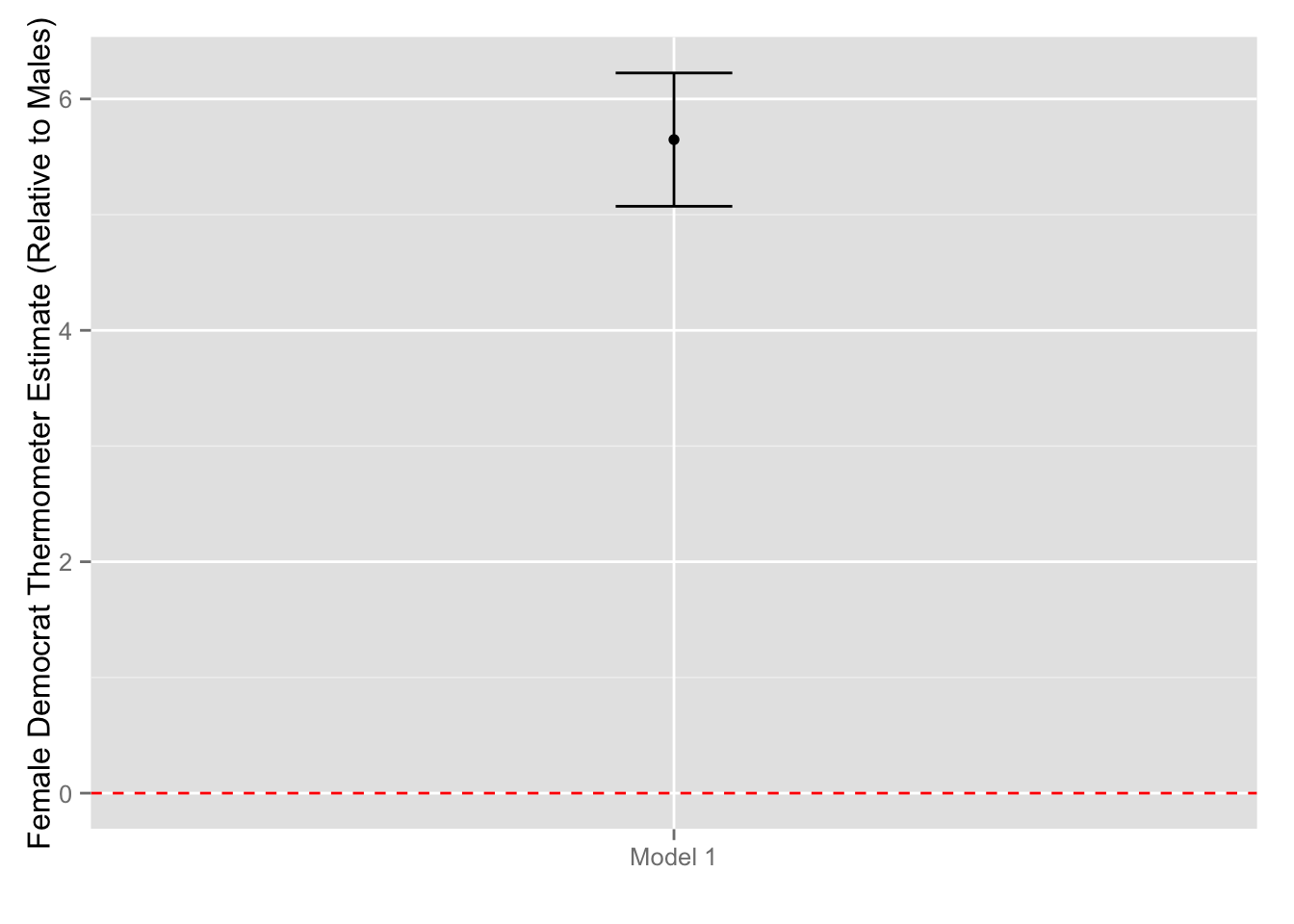1.1.2 Graphing Approach 2

Here’s a different approach using R’s predict() function. Before you use this command, however, ask yourself the following:

• Given the estimates from the model, what is the “predicted” feeling rating among women?
• Given the estimates from the model, what is the “predicted” feeling rating among men?
• What is the predicted gap between women and men and how does that relate to the graph above?
pred1 <- predict(lm1,
newdata = data.frame(gender = c("Male", "Female")),
se.fit = T,
interval = "confidence")

# The result is a list with estimates, 95% CIs, and other info:
pred1
## \$fit
##     fit   lwr   upr
## 1 58.99 58.56 59.42
## 2 64.64 64.25 65.02
##
## \$se.fit
##      1      2
## 0.2190 0.1961
##
## \$df
##  26403
##
## \$residual.scale
##  23.74
# Extract part of the list with estimates and confidence interval, and add gender info:
pred1 <- data.frame(pred1\$fit, gender = c("Male", "Female"))

ggplot(pred1, aes(x = gender, y = fit, color = gender)) +
geom_point() +
geom_errorbar(aes(ymin = lwr, ymax = upr), width = 0.1) +
xlab("") +
ylab("Predicted Democrat Thermometer")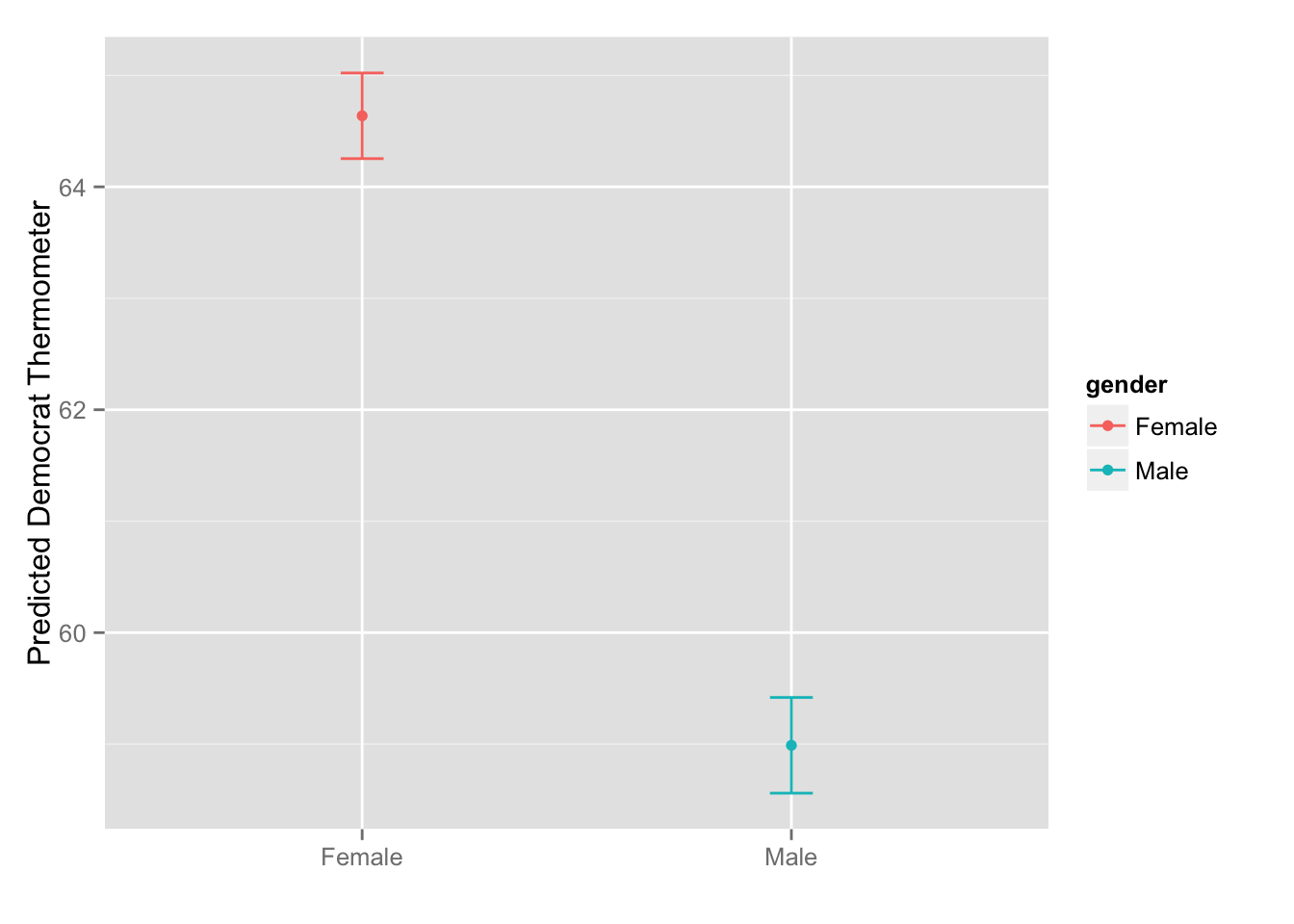The newdata argument of the predict() function lets you supply the values for which you want a prediction. You have to use the same variable name(s) as those of the variable(s) included in lm().

1.2 Multivariate model

Let’s add income and age to the model. Income in particular may be a confounder here, since women have lower income and income is negatively correlated with support for the democratic party.

levels(anes\$income) <- NA
levels(anes\$income) <- with(anes, substr(levels(income), 4, nchar(levels(income))))
anes\$age[anes\$age == 0] <- NA

lm2 <- lm(demtherm ~ gender + age + income, data = anes)
display(lm2)
## lm(formula = demtherm ~ gender + age + income, data = anes)
##                            coef.est coef.se
## (Intercept)                 64.00     0.59
## genderFemale                 3.89     0.30
## age                          0.08     0.01
## income17 to 33 percentile   -3.99     0.51
## income34 to 67 percentile   -8.50     0.45
## income68 to 95 percentile  -12.48     0.47
## income96 to 100 percentile -17.94     0.74
## ---
## n = 23686, k = 7
## residual sd = 22.92, R-Squared = 0.06

** Exercise**: Interpret each of the coefficient estimates from this model.

1.2.1 Graphing Approach 1

Again, we can graph the estimate on female. For comparison, let’s put it next to the estimate from the bivariate model above.

ci2 <- confint(lm2, level = 0.95)[2, ]

est2 <- data.frame(est = coef(lm2),
lb = ci2,
ub = ci2,
model = "Model 2")

est <- rbind(est1, est2)   #combine with estimates from first model

ggplot(est, aes(x = model, y = est)) +
geom_point() +
geom_errorbar(aes(ymin = lb, ymax = ub), width = 0.1) +
geom_hline(yintercept = 0, lty = 2, color = "red") +
xlab("") +
ylab("Female Democrat Thermometer Estimate (Relative to Males)")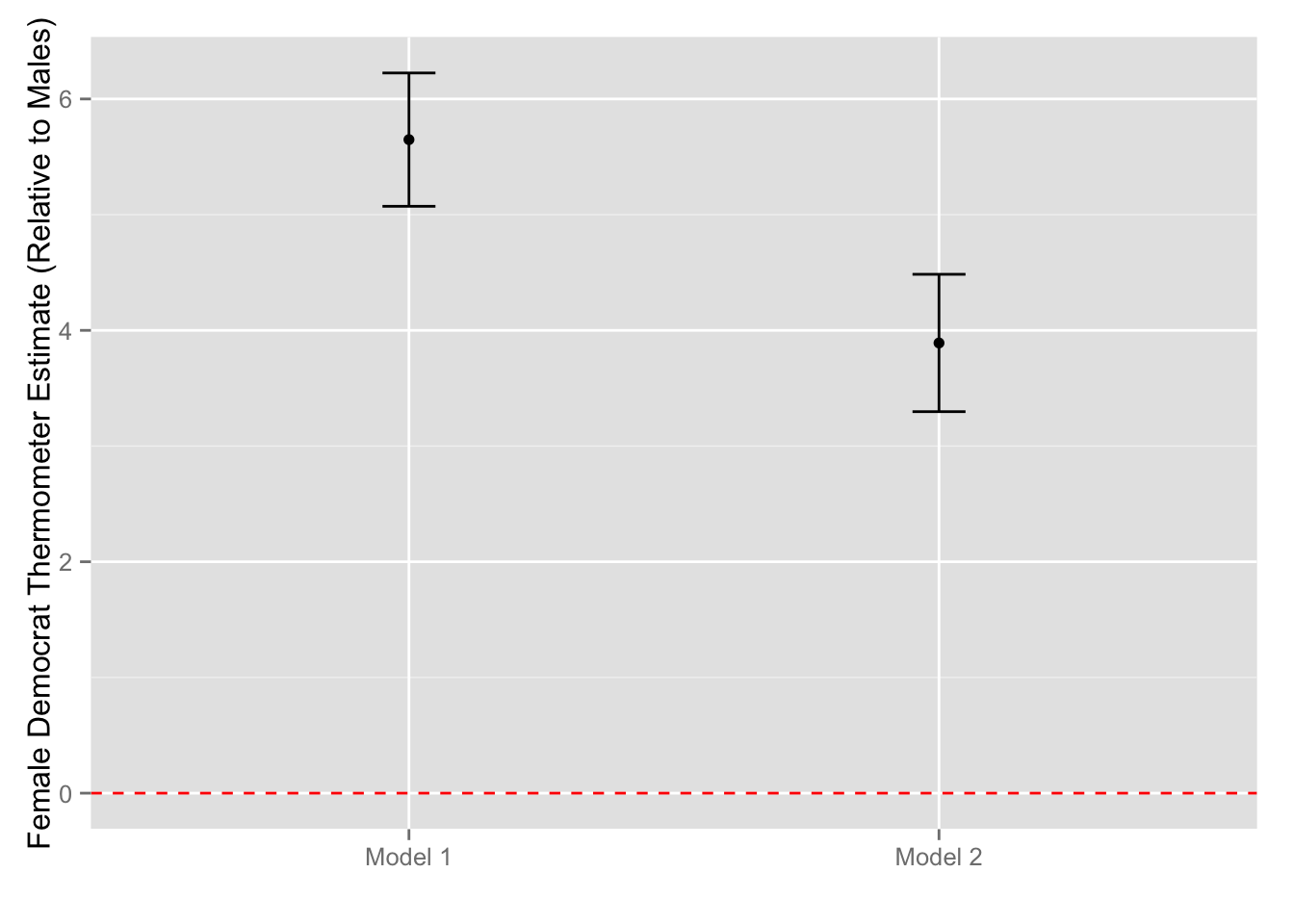1.2.2 Graphing Approach 2

We can also graph the predicted values for males and females for “average” values of the covariates.

Mean age is 46 years:

mean(anes\$age, na.rm = T)
##  45.84

The most common income category is “34 to 67 percentile”:

prop.table(table(anes\$income))
##
##   0 to 16 percentile  17 to 33 percentile  34 to 67 percentile
##              0.17108              0.16785              0.32710
##  68 to 95 percentile 96 to 100 percentile
##              0.27925              0.05473

So let’s use these (constant) values for each of our covariates, while varying gender.

** Exercise**: Before you use the predict() function, calculate by hand the predicted values for males and females at the average values of age and income.

Now let’s graph the predicted values:

newdta <- data.frame(gender = c("Male", "Female"),
age = rep(mean(anes\$age, na.rm = T), 2),
income = rep("34 to 67 percentile", 2))

pred2 <- predict(lm2,
newdata = newdta,
se.fit = T,
interval = "confidence")

pred2 <- data.frame(pred2\$fit, gender = c("Male", "Female"))

ggplot(pred2, aes(x = gender, y = fit, color = gender)) +
geom_point() +
geom_errorbar(aes(ymin = lwr, ymax = upr), width = 0.1) +
xlab("\nNote: Estimates condition on income and age") +
ylab("Predicted Democrat Thermometer")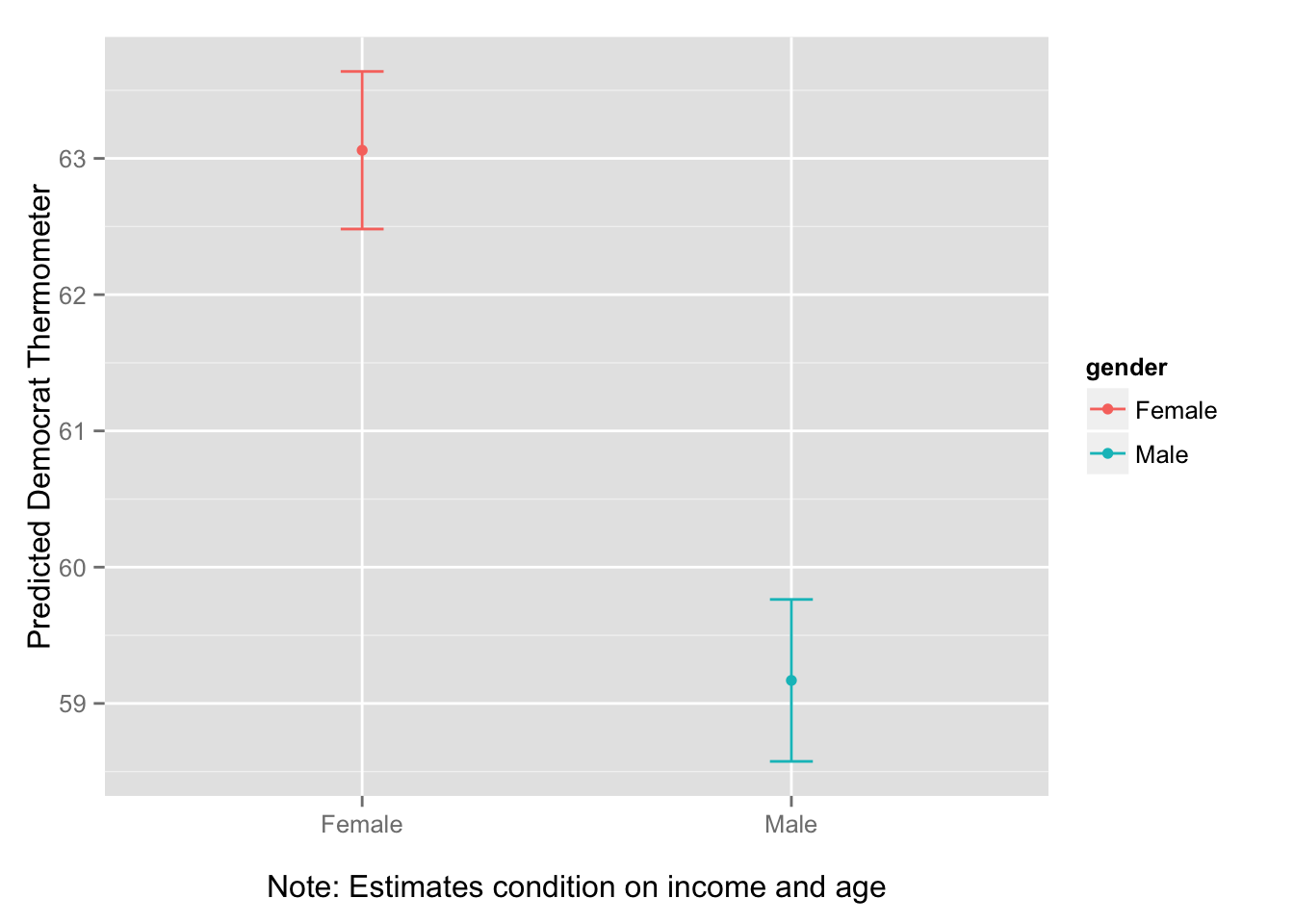1.3 Relationship over time

The previous models group respondents from all ANES surveys starting in 1978. (The democratic rating was not asked before 1978, and lm() automatically excludes missing observations.)

Let’s examine the relationship between gender and the democratic rating over time, while still conditioning on age and income.

First run a separate regression for each year starting in 1978. I use dlply() here: it takes a data frame, splits it by year, and returns a list with the model estimates for each year:

anes_sub <- subset(anes, year >= 1978 & year != 2002)

lm3 <- dlply(anes_sub, .(year), function(x) {
lm(demtherm ~ gender + age + income, data = x)
})

display(lm3\$"1990")   #estimates for year 1990
## lm(formula = demtherm ~ gender + age + income, data = x)
##                            coef.est coef.se
## (Intercept)                 61.10     1.98
## genderFemale                 4.54     1.05
## age                          0.11     0.03
## income17 to 33 percentile   -1.16     1.76
## income34 to 67 percentile   -4.33     1.55
## income68 to 95 percentile   -8.66     1.56
## income96 to 100 percentile -20.20     2.73
## ---
## n = 1792, k = 7
## residual sd = 21.68, R-Squared = 0.07

Now grab the estimate on gender as well as the associated 95% confidence interval for each year. Now I use ldply: it takes a list and returns a data frame:

est_time <- ldply(lm3, function(x) {
c(coef(x), confint(x)[2, ])
})

names(est_time) <- c("year", "est", "lb", "ub")
est_time
##    year   est      lb    ub
## 1  1978 3.485  1.5872 5.382
## 2  1980 1.668 -0.7725 4.108
## 3  1982 3.407  1.0232 5.792
## 4  1984 2.177  0.2555 4.098
## 5  1986 3.763  1.7877 5.739
## 6  1988 4.140  1.9031 6.376
## 7  1990 4.544  2.4844 6.603
## 8  1992 4.107  2.2672 5.947
## 9  1994 3.367  1.1190 5.615
## 10 1996 4.329  1.9330 6.725
## 11 1998 5.192  2.5626 7.822
## 12 2000 4.728  2.2240 7.231
## 13 2004 3.246  0.3541 6.138
## 14 2008 5.180  3.0823 7.278

Now graph these estimates:

ggplot(est_time, aes(x = year, y = est)) +
geom_point() +
geom_errorbar(aes(ymin = lb, ymax = ub), width = 0.3) +
geom_hline(yintercept = 0, lty = 2, color = "red") +
xlab("") +
ylab("Female Democrat Thermometer Estimate (Relative to Males)")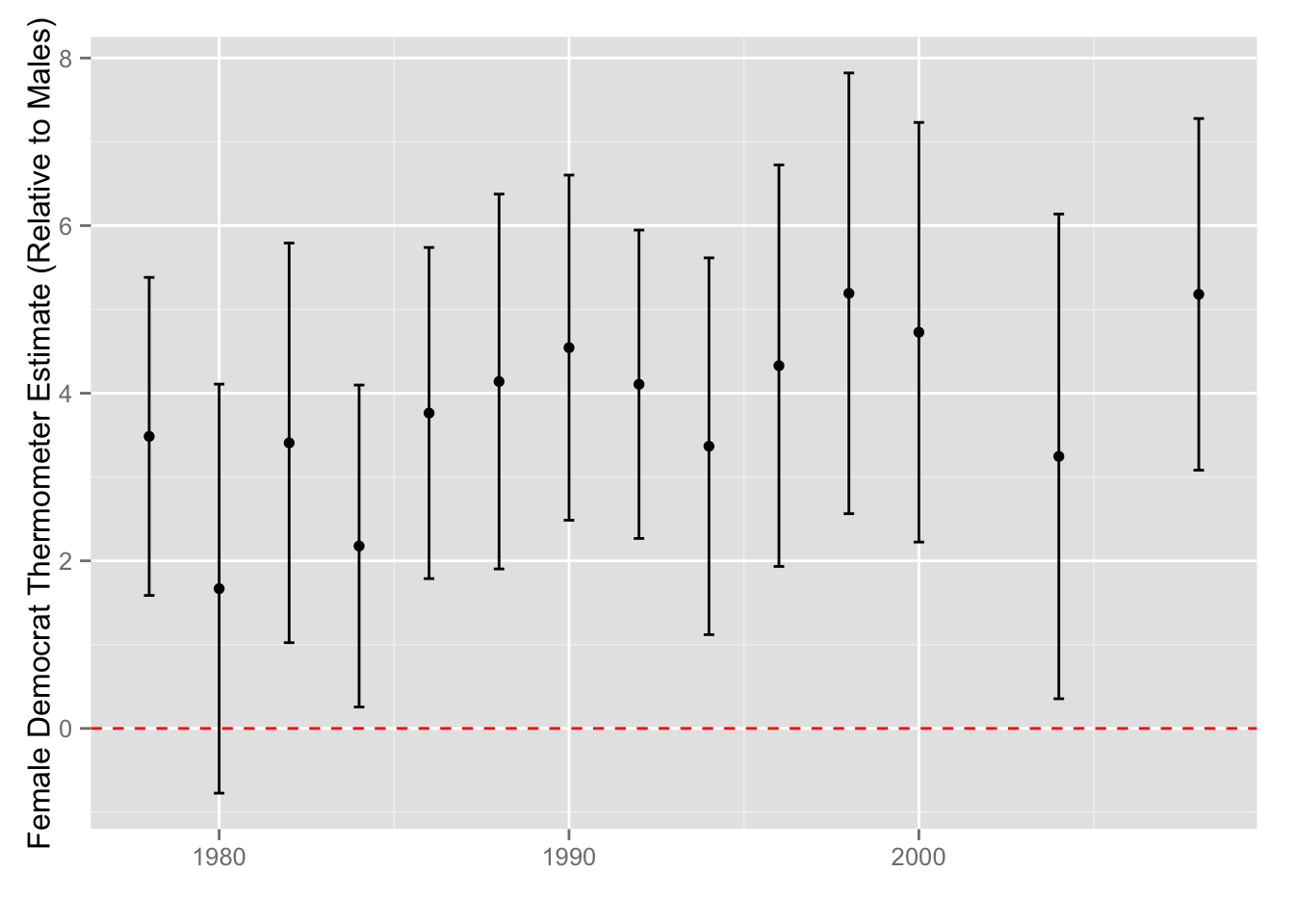Again, we can graph the predicted values instead:

pred_time <- ldply(lm3, function(x) {
predict(x, newdata = newdta, se.fit = T, interval = "confidence")\$fit
})

pred_time\$gender <- rep(c("Male", "Female"), length(pred_time)/2)

ggplot(pred_time, aes(x = year, y = fit, color = gender)) +
geom_point() +
geom_line() +
geom_ribbon(aes(ymax = upr, ymin = lwr, fill = gender),
alpha = 0.15, color = NA) +
xlab("\nNote: Income and age held at average values") +
ylab("Predicted Democrat Thermometer")